# The HPLMIXED Procedure

### PROC HPLMIXED Statement

PROC HPLMIXED <options> ;

The PROC HPLMIXED statement invokes the procedure. Table 8.2 summarizes important options in the PROC HPLMIXED statement by function. These and other options in the PROC HPLMIXED statement are then described fully in alphabetical order.

Table 8.2: PROC HPLMIXED Statement Options

Option

Description

Basic Options

Specifies the input data set

Specifies the estimation method

Limits the length of effect names

Computes the best linear unbiased prediction

Options Related to Output

Suppresses the Class Level Information table completely or in parts

Specifies the maximum levels of CLASS variables to print

Displays the rank of design matrix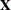Optimization Options

Tunes an absolute function convergence criterion

Tunes an absolute function difference convergence criterion

Tunes the absolute gradient convergence criterion

Tunes the relative function convergence criterion

Tunes the relative gradient convergence criterion

Chooses the maximum number of iterations in any optimization

Specifies the maximum number of function evaluations in any optimization

Specifies the upper limit on seconds of CPU time for any optimization

Specifies the minimum number of iterations in any optimization

Selects the optimization technique

Tunes the relative parameter convergence criterion

You can specify the following options in the PROC HPLMIXED statement.

ABSCONV=r

specifies an absolute function convergence criterion. For minimization, termination requires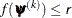, where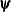is the vector of parameters in the optimization and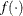is the objective function. The default value of r is the negative square root of the largest double-precision value, which serves only as a protection against overflows.

ABSFCONV=r

specifies an absolute function difference convergence criterion. For all techniques except Nelder–Mead simplex (NMSIMP), termination requires a small change of the function value in successive iterations: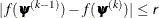Here,denotes the vector of parameters that participate in the optimization andis the objective function. The same formula is used for the NMSIMP technique, but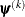is defined as the vertex with the lowest function value and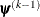is defined as the vertex with the highest function value in the simplex. The default value is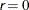.

ABSGCONV=r

specifies an absolute gradient convergence criterion. Termination requires the maximum absolute gradient element to be small: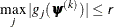Here,denotes the vector of parameters that participate in the optimization and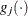is the gradient of the objective function with respect to theparameter. This criterion is not used by the NMSIMP technique. The default value is r=1E–5.

BLUP<(suboptions)>

requests that best linear unbiased predictions (BLUPs) for the random effects be displayed. To use this option, you must also use the PARMS statement to specify fixed values for the covariance parameters, which means that the NOITER option in the PARMS statement will be implied by the BLUP option. Also, the iterations in the ODS Table IterHistory will refer to iterations used to compute the BLUP rather than optimization iterations.

The BLUP solution might be sensitive to the order of observations, and hence to how the data are distributed on the grid. If there are multiple measures of a repeated effect, then the BLUP solution is not unique. If the order of these multiple measures on the grid differs for different runs, then different BLUP solutions will result.

You can specify the following suboptions:

ITPRINT=number

specifies that the iteration history be displayed after every number of iterations. The default value is 10, which means the procedure displays the iteration history for every 10 iterations.

MAXITER=number

specifies the maximum number of iterations allowed. The default value is the number of parameters in the BLUP option plus 2.

TOL=number

specifies the tolerance value. The default value is the square root of machine precision.

DATA=SAS-data-set

names the SAS data set to be used as the input data set. The default is the most recently created data set.

FCONV=r

specifies a relative function convergence criterion. For all techniques except NMSIMP, termination requires a small relative change of the function value in successive iterations,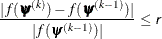Here,denotes the vector of parameters that participate in the optimization andis the objective function. The same formula is used for the NMSIMP technique, butis defined as the vertex with the lowest function value andis defined as the vertex with the highest function value in the simplex.

The default is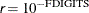, where FDIGITS is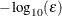andis the machine precision.

GCONV=r

specifies a relative gradient convergence criterion. For all techniques except CONGRA and NMSIMP, termination requires that the normalized predicted function reduction be small,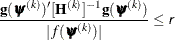Here,denotes the vector of parameters that participate in the optimization,is the objective function, and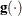is the gradient. For the CONGRA technique (where a reliable Hessian estimateis not available), the following criterion is used: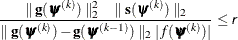This criterion is not used by the NMSIMP technique. The default value is r=1E–8.

MAXCLPRINT=number

specifies the maximum levels of CLASS variables to print in the ODS table ClassLevels. The default value is 20. MAXCLPRINT=0 enables you to print all levels of each CLASS variable. However, the option NOCLPRINT takes precedence over MAXCLPRINT.

MAXFUNC=n

specifies the maximum numberof function calls in the optimization process. The default values are as follows, depending on the optimization technique:

• TRUREG, NRRIDG, NEWRAP: 125

• QUANEW, DBLDOG: 500

• CONGRA: 1,000

• NMSIMP: 3,000

The optimization can terminate only after completing a full iteration. Therefore, the number of function calls that are actually performed can exceed n. You can choose the optimization technique with the TECHNIQUE= option.

MAXITER=n

specifies the maximum numberof iterations in the optimization process. The default values are as follows, depending on the optimization technique:

• TRUREG, NRRIDG, NEWRAP: 50

• QUANEW, DBLDOG: 200

• CONGRA: 400

• NMSIMP: 1,000

These default values also apply whenis specified as a missing value. You can choose the optimization technique with the TECHNIQUE= option.

MAXTIME=r

specifies an upper limit ofseconds of CPU time for the optimization process. The default value is the largest floating-point double representation of your computer. The time specified by the MAXTIME= option is checked only once at the end of each iteration. Therefore, the actual running time can be longer than r.

METHOD=REML
METHOD=ML

specifies the estimation method for the covariance parameters. METHOD=REML performs residual (restricted) maximum likelihood; it is the default method. METHOD=ML performs maximum likelihood.

MINITER=n

specifies the minimum number of iterations. The default value is 0. If you request more iterations than are actually needed for convergence to a stationary point, the optimization algorithms can behave strangely. For example, the effect of rounding errors can prevent the algorithm from continuing for the required number of iterations.

NAMELEN=number

specifies the length to which long effect names are shortened. The minimum value is 20, which is also the default.

NOCLPRINT<=number>

suppresses the display of the Class Level Information table if you do not specify number. If you specify number, the values of the classification variables are displayed for only those variables whose number of levels is less than number. Specifying a number helps to reduce the size of the Class Level Information table if some classification variables have a large number of levels.

NOPRINT

suppresses the generation of ODS output.

RANKS

displays the rank of design matrix.

SINGCHOL=number

tunes the singularity criterion in Cholesky decompositions. The default is 1E4 times the machine epsilon; this product is approximately 1E–12 on most computers.

SINGSWEEP=number

tunes the singularity criterion for sweep operations. The default is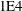times the machine epsilon; this product is approximately 1E–12 on most computers.

SINGULAR=number

tunes the general singularity criterion applied by the HPLMIXED procedure in sweeps and inversions. The default istimes the machine epsilon; this product is approximately 1E–12 on most computers.

SPARSE

specifies the sparse technique is used together with BLUP option. In general, this option makes PROC HPLMIXED to be more efficient in terms of timing and memory. It supports the following covariance types in RANDOM statement: VC, AR, CS, UC, UCH, CSH, UN CHOL and TOEP(1).

TECHNIQUE=keyword

specifies the optimization technique for obtaining maximum likelihood estimates. You can specify any of the following keywords:

CONGRA

performs a conjugate-gradient optimization.

DBLDOG

performs a version of double-dogleg optimization.

NEWRAP

performs a Newton-Raphson optimization combining a line-search algorithm with ridging.

NMSIMP

performs a Nelder-Mead simplex optimization.

NONE

performs no optimization.

NRRIDG

performs a Newton-Raphson optimization with ridging.

QUANEW

performs a dual quasi-Newton optimization.

TRUREG

performs a trust-region optimization.

The default value is TECHNIQUE=NRRIDG.

XCONV=r

specifies the relative parameter convergence criterion:

• For all techniques except NMSIMP, termination requires a small relative parameter change in subsequent iterations: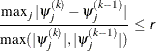• For the NMSIMP technique, the same formula is used, but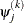is defined as the vertex with the lowest function value and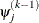is defined as the vertex with the highest function value in the simplex.

The default value is r = 1E–8 for the NMSIMP technique and r = 0 otherwise.